NCERT Solutions for Class 9 Maths Chapter 7 Heron’s Formula Ex 7.1 are part of NCERT Solutions for Class 9 Maths. Here we have given NCERT Solutions for Class 9 Maths Chapter 7 Heron’s Formula Ex 7.1

 Board CBSE Textbook NCERT Class Class 9 Subject Maths Chapter Chapter 7 Chapter Name Heron’s Formula Exercise Ex 7.1 Number of Questions Solved 6 Category NCERT Solutions

## NCERT Solutions for Class 9 Maths Chapter 7 Heron’s Formula Ex 7.1

Question 1.
A traffic signal board, indicating ‘SCHOOL AHEAD’, is an equilateral triangle with side a. Find the area of the signal board, using Heron’s formula.If its perimeter is 180 cm, what will be the area of the signal board?
Solution:
We know that, an equilateral triangle has equal sides. So, all sides are equal to a.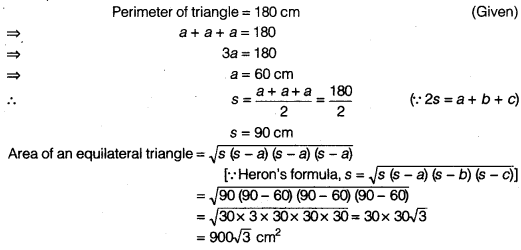Question 2.
The triangular side walls of a flyover have been used for advertisements. The sides of the walls are 122 m, 22 m and 120 m (see figure). The advertisements yield an earning of ₹5000 per m2 per year. A company hired one of its walls for 3 months. How much rent did it pay?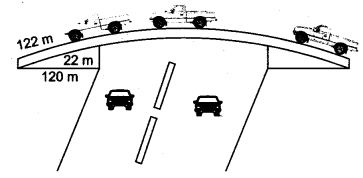Solution:
Leta = 122m,
b = 22m
c = 120m
We have, b2 + c2 = (22)2 + (120)2 = 484 + 14400 = 14884= (122)2 = a2
Hence, the side walls are in right triangled shape.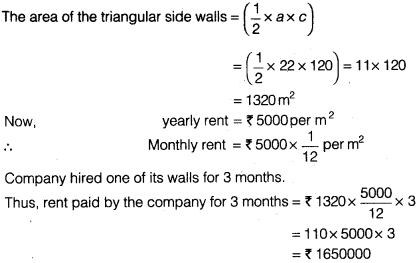Question 3.
There is a slide in a park. One of its side Company hired one of its walls for 3 months.walls has been painted in some colour with a message “KEEP THE PARK GREEN AND CLEAN” (see figure). If the sides of the wall are 15 m, 11 m and 6m, find the area painted in colour.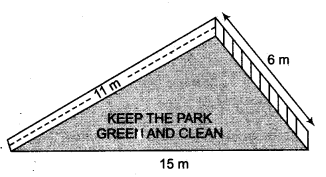Solution:
The given figure formed a triangle whose sides are
a = 15m
b = 11m,
c = 6m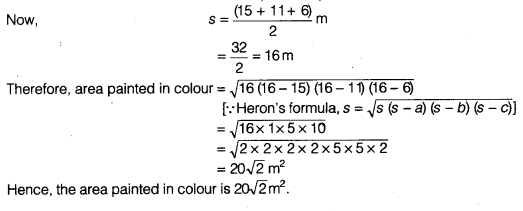Question 4.
Find the area of a triangle two sides of which are 18 cm and 10 cm and the perimeter is 42 cm.
Solution:
Solution Let the sides of a triangle, a = 18cm b = 10 cm and c
We have, perimeter = 42 cm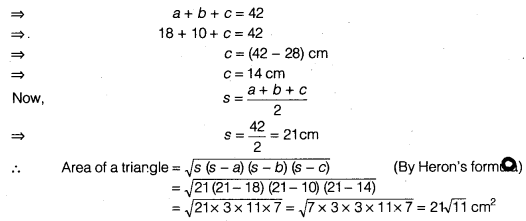Question 5.
Sides of a triangle are in the ratio of 12 : 17 : 25 and its perimeter is 540 cm. Find its area.
Solution:
Suppose that the sides in cm, are 12x, 17x and 25x.
Then, we know that 12x + 17x + 25x = 540 (Perimeter of triangle)
⇒ 54x = 540
⇒ x=10
So, the sides of the triangle are 12 x 10 cm, 17 x 10 cm 25 x 10 cm
i.e., 120 cm, 170 cm, 250 cm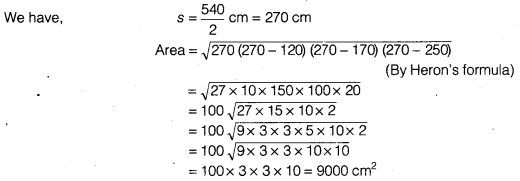Question 6.
An isosceles triangle has perimeter 30 cm and each of the equal sides is 12 cm. Find the area of the triangle.
Solution: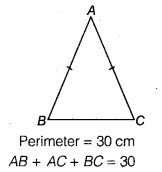Let in isosceles ∆ ABC,
AB = AC = 12 cm (Given)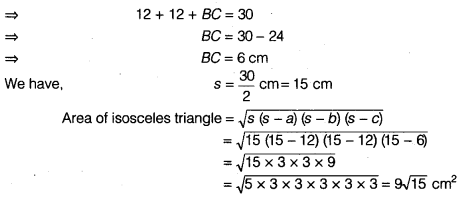We hope the NCERT Solutions for Class 9 Maths Chapter 7 Heron’s Formula Ex 7.1 help you. If you have any query regarding NCERT Solutions for Class 9 Maths Chapter 7 Heron’s Formula Ex 7.1, drop a comment below and we will get back to you at the earliest.